• 用matlab 怎么画函数曲线图

千次阅读 2021-04-18 15:06:47
下面我将介绍matlab画函数图形的方法，以在同一张图上画出y1=sin(x)和y2=sin^2(x)的图形例。工具/原料matlab1方法/步骤输入自变量：画已知的函数图形，向量的方式表示自变量比较方便。代码x=linspace(0,8); ...

matlab的数据处理能力很强大，这款软件还能绘制各种函数图形。下面我将介绍用matlab画函数图形的方法，以在同一张图上画出y1=sin(x)和y2=sin^2(x)的图形为例。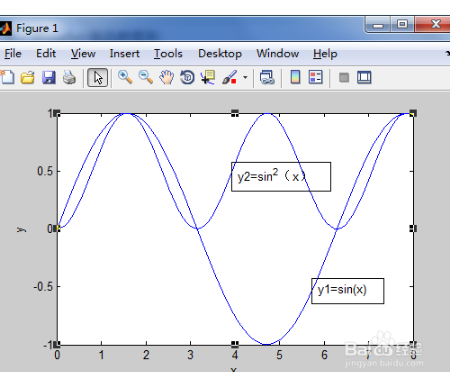工具/原料

matlab

1方法/步骤

输入自变量：

画已知的函数图形，用向量的方式表示自变量比较方便。代码为

x=linspace(0,8);                  % 用向量形式创建x。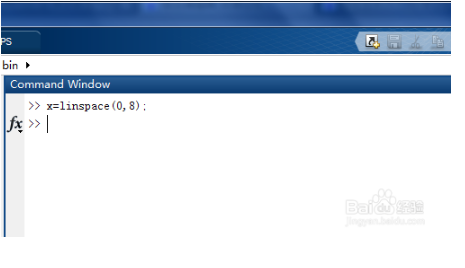2输入因变量表达式：

y1=sin(x);                       % 向量y1的值为x坐标上某一x的s i n ( 2 x )值。

y2=(sin(x)).^2 ;               % 向量y2的值为( s i n ( x ) ) .^2,注意别忘记一点”.“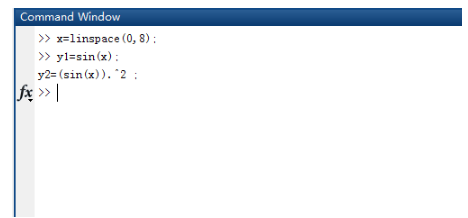3 使用plot命令画图：

plot(x,y1)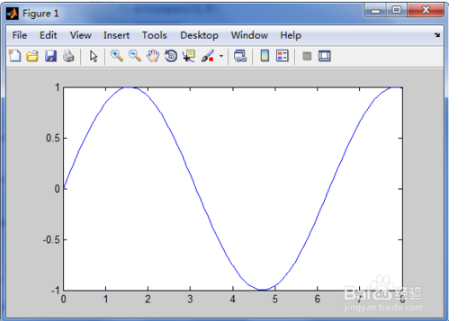4按回车，则画出y1=sin(x)的图形。

如果这时直接继续画y2=sin^2(x)的图形，则在另一张图中画，为了在同一张图中画两个函数图，输入下面代码：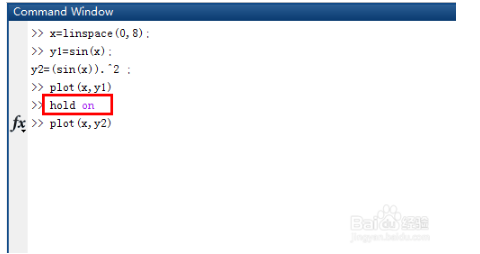hold on

5再输入绘图命令：

plot(x,y2)

按回车，则在同一张图上画出了这两个函数的图形。可以通过菜单栏中的insert添加坐标名称，文本等。

其他函数的画法类似！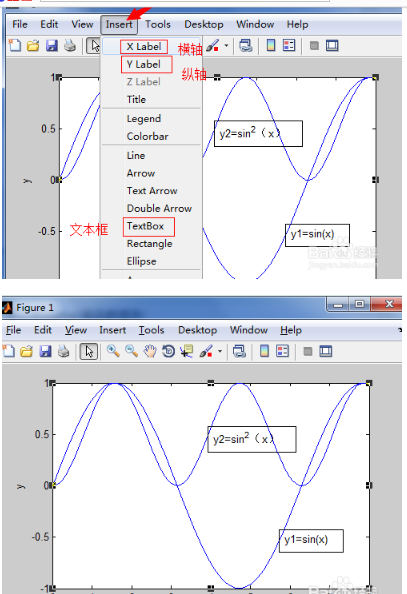展开全文• 为什么MATLAB多项式拟合得到的函数,再用函数去作图时两者的曲线会不一样呢?2018-9-16来自ip:10.170.179.125的网友咨询浏览量:135问题补充：为什么MATLAB多项式拟合得到的函数,再用函数去作图时两者的曲线会...

为什么我用MATLAB多项式拟合得到的函数,再用函数去作图时两者的曲线会不一样呢?

2018-9-16来自ip:10.170.179.125的网友咨询

浏览量:135

问题补充：

为什么我用MATLAB多项式拟合得到的函数,再用函数去作图时两者的曲线会不一样呢?

clear all

x0=[0 0.5 1 1.5 2 2.5 3 7 8 9 10 11.82 13 14 15 16 17.73 19 20 21 22 23.64 26 27 28 29 29.55];

y0=[1.892e9 1.89e9 1.87e9 1.86e9 1.85e9 1.84e9 1.83e9 3e5 3e5 3.1e5 3e5 2.9e5 2.4e5 2.3e5 2e5 2.2e5 2e5 1.85e5 1.8e5 1.78e5 1.75e5 1.7e5 1.62e5 1.65e5 1.6e5 1.55e5 1.5e5];

p3=polyfit(x0,y0,18);

vpa(poly2sym(p3),5)

x=0:0.1:29.6;

y1=polyval(p3,x);

>> plot(x,y1,x0,y0,'o')

grid on

得到如下函数：

y=1.604*10^(-9)*x^18 - 4.4275*10^(-7)*x^17 + 0.000056159*x^16 - 0.0043377*x^15 + 0.22791*x^14 - 8.6173*x^13 + 241.87*x^12 - 5123.9*x^11 + 82462.0*x^10 - 1.0064*10^6*x^9 + 9.2252*10^6*x^8 - 6.2331*10^7*x^7 + 3.0125*10^8*x^6 - 9.9516*10^8*x^5 + 2.1031*10^9*x^4 - 2.5932*10^9*x^3 + 1.6075*10^9*x^2 - 3.7944*10^8*x + 1.8927*10^9

我再输入

clear all

x=0:0.1:30;

y=1.604e-9*x.^18 - 4.4275e-7*x.^17 + 0.000056159*x.^16 - 0.0043377*x.^15 + 0.22791*x.^14 - 8.6173*x.^13 + 241.87*x.^12 - 5123.9*x.^11 + 82462.0*x.^10 - 1.0064e6*x.^9 + 9.2252e6*x.^8 - 6.2331e7*x.^7 + 3.0125e8*x.^6 - 9.9516e8*x.^5 + 2.1031e9*x.^4 - 2.5932e9*x.^3 + 1.6075e9*x.^2 - 3.7944e8*x+ 1.8927e9;

plot(x,y)

为什么曲线会不一样,我仔细看过只有曲线前一部分会是相同的大概在x小于5.5内是相同的,之后就完全不一样了.请教给位了.实在不明白为什么了.

主要是这个得到的曲线，一部分小于0，我是希望把常数项再变大一些，使得曲线完全在x轴上方。哪知道画出来的曲线完全不是那么回事。

展开全文• 如何用matlab画出函数曲线

千次阅读 2021-04-19 00:38:11
使用matlab绘制函数曲2113线的方法和操作步5261骤如下：1、首先，使用matlabc打开命令行窗口，然后4102直接输入相1653关内容，如下图所示。2、其次，完成上述步骤后，请根据实际情况设置图示代码，如下图所示。3、...

使用matlab绘制函数曲2113线的方法和操作步5261骤如下：1、首先，使用matlabc打开命令行窗口，然后4102直接输入相1653关内容，如下图所示。2、其次，完成上述步骤后，请根据实际情况设置图示代码，如下图所示。3、接着，完成上述步骤后，需要填写注释信息，如下图所示。4、最后，完成上述步骤后，将获得相应的效果图，函数曲线就绘制完成了，如下图所示www.mh456.com防采集。

1、用2113matlabc打开命令行窗口，直接输入相关的5261内容。

>>x=-2*pi:0.1*pi:2*pi;y=sin(x);plot(x,y) grid on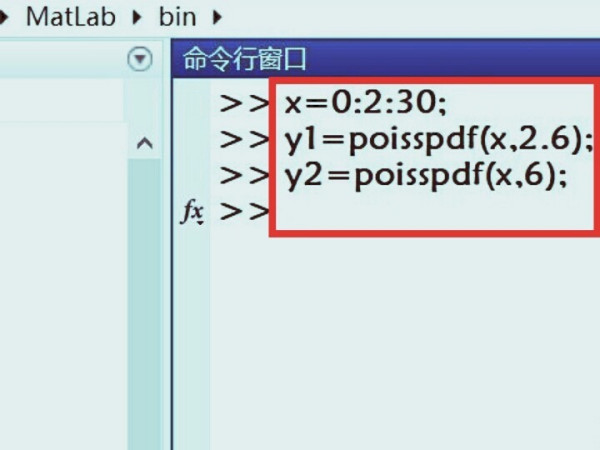2、下一步如果没4102问题，就根据实际情1653况来设置图示的代码。

用matlab画分段函数曲面图，可以先自定义分段函数p=func(x1，x2)，再用meshgrid()函数将x1,x2网格化，再求出p值，最后用mesh(x1，x2，p)命令绘出其曲面图。编程后运行可达到如下图形。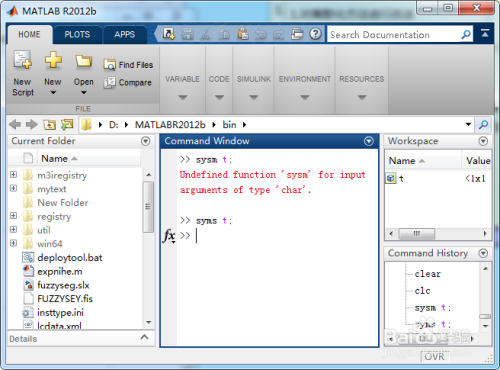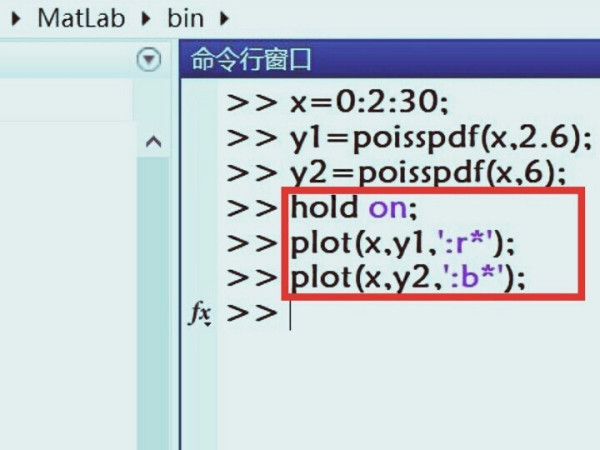3、这个时候通过确定操作以后，需要填写注释的信息。

x=0:0.01:1;y=sqrt(1-x.^2);plot(x,y);原来的函数 x0=0.6;y0=0.8;曲线上的一点 hold on;在画完函数虚线之后保持曲线图 plot([0 x0],[y0 y0],'r-');画两个虚线 plot([x0 x0],[0 y0],'r-');hold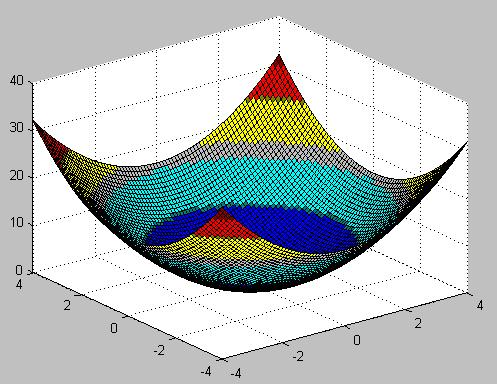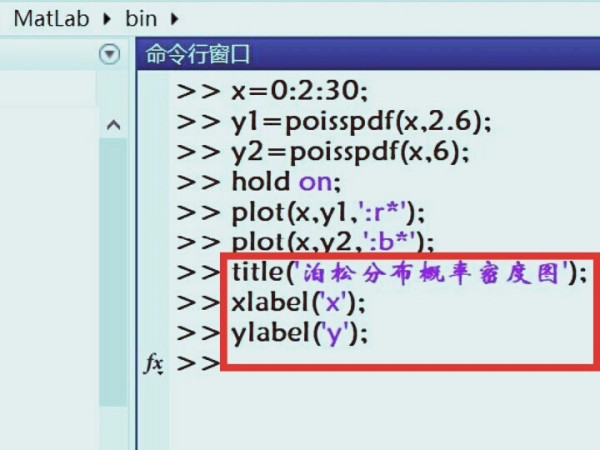4、这样一来会得到对应的效果图，即可画出函数曲线了。

拟合步骤: 1、求(获)得一系列x,y对应值 x=[.] y=[.] 2、根据画出的曲线,设定拟合函数 fun=inline('a(1)+a(2)*exp(a(3)*x','a','x') 3、初定x0的初值 x0=[0 0 0] 4、用拟合函数求出拟合系数 a=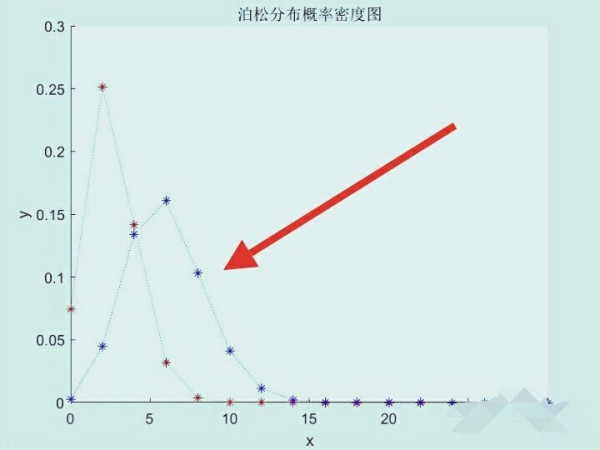2113　Matlab画图的命令一般用plot，其基本原理是把散点5261连起来构成图像。所以，画二维图4102时，plot的参数1653中需要有x，y轴的数据

下面，给出来几个例子。

1.画sin(x)的函数图像      x = 0:pi/100:2*pi;y = sin(x);figure % opens new figure windowplot(x,y)

结果如下：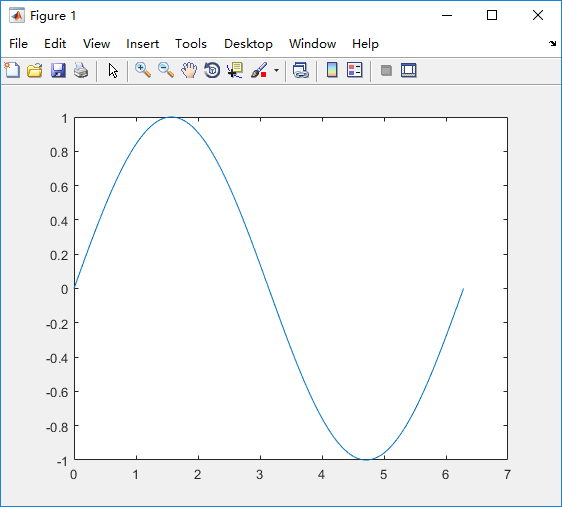2. 将多个图像画在一张图中x = 0:pi/100:2*pi;y1 = sin(x);y2 = sin(x-0.25);y3 = sin(x-0.5);figureplot(x,y1,x,y2,'--',x,y3,':')legend('sin(x)','sin(x-0.25)','sin(x-0.5)');

结果如下：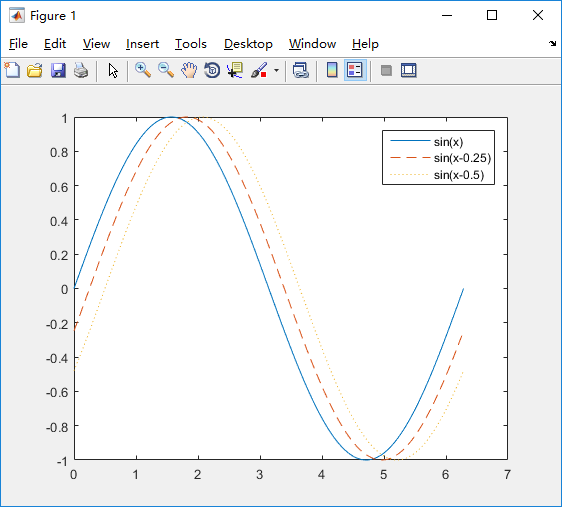你要想了解更多的用法，可以在Matlab的命令窗口中输入：doc plot

来查看详细的信息。本回答被网友采纳

matlab有很多画图函数，比如二维的有plot()，三维的有其他，可根据你画的函数来选择作图函数，选择合适的工具函数会让你事半功倍。

内容来自www.mh456.com请勿采集。

展开全文• 如下图就是在Excel中绘制的正、余弦函数图像：一、准备数据1、首先要根据函数表达式准备一组数据，然后利用该数据在图表中绘制出函数图像。本例中将数据放置在区域A:C列中，其中A列中的数值函数自变量的值，在A列...

朋友们一定会遇到画函数曲线的问题吧！如果想快速准确地绘制一条函数曲线，可以借助EXCEL的图表功能，它能使你画的曲线既标准又漂亮。还可以将多个函数图像放入同一图表中进行对比。如下图就是在Excel中绘制的正、余弦函数图像：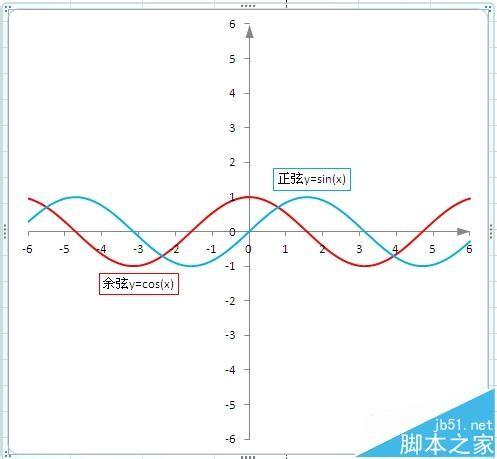一、准备数据

1、首先要根据函数表达式准备一组数据，然后利用该数据在图表中绘制出函数图像。本例中将数据放置在区域A:C列中，其中A列中的数值为函数自变量的值，在A列的A1单元格输入"X="，表明这是自变量，A2、A3及以下的单元格内逐次从小到大输入自变量的各个值，实际输入的时候，通常应用等差数列输入法。如何确定自变量的初始值，取值范围，数据点之间的步长是多少，这是要根据函数的具体特点来判断。如果想很快查到函数的极值或看出其发展趋势，给出的数据点也不一定非得是等差的，可以根据需要任意给定。如图，我作图用的取值范围为[-6,6]，数值之间的间隔为“0.1”。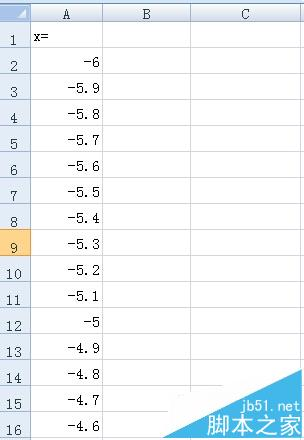2、B列和C列为两个函数应变量的值。在B列的B1单元格输入函数式的一般书面表达形式，y=sin(x)，可以加一些说明性字符；在B2单元格中根据函数表达式输入公式“=SIN(A2)” 然后选中B2单元格，填充下拉。同样在C列输入函数达形式和公式。如图，这样数据就准备好了。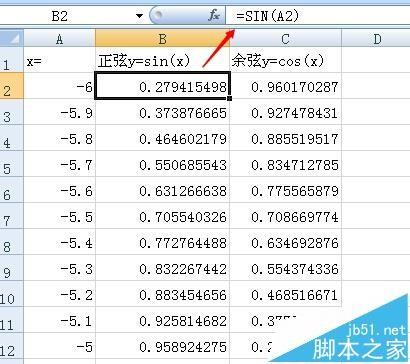二、绘制函数图像

1、在功能区中选择“插入”选项卡，在“图表”组中依次单击“散点图→带平滑线的散点图”。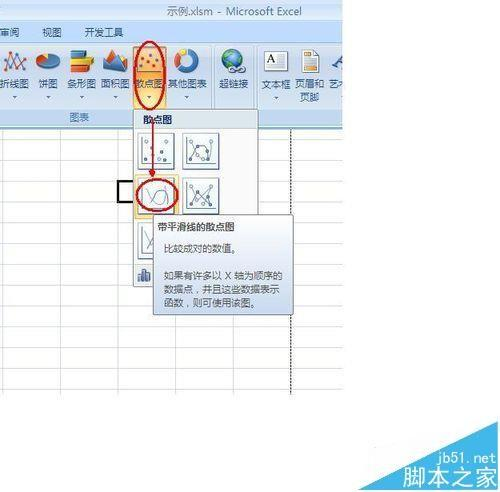2、Excel将插入如下图所示的图表区，并在功能区中增加图表工具的“设计”、“布局”“格式”选项卡。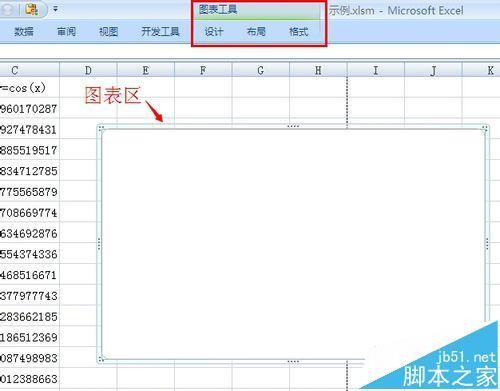3、首先要选择图表的数据，鼠标在图表区内右击，在弹出的快捷菜单中选择“选择数据”。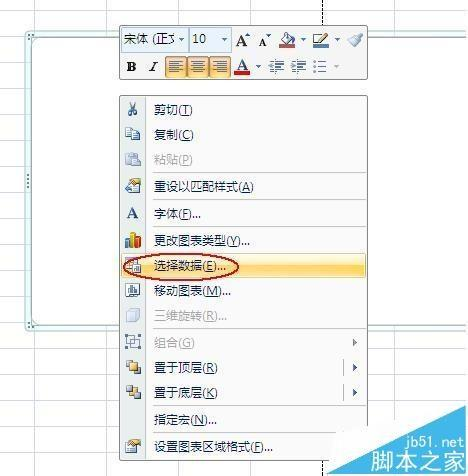4、在弹出的“选择数据源”对话框中选择“添加”，弹出“编辑数据系列”对话框，如图，选择我们准备好的数据，点“确定”。这样正弦函数图像就添加上了，同样把余弦函数也添加上。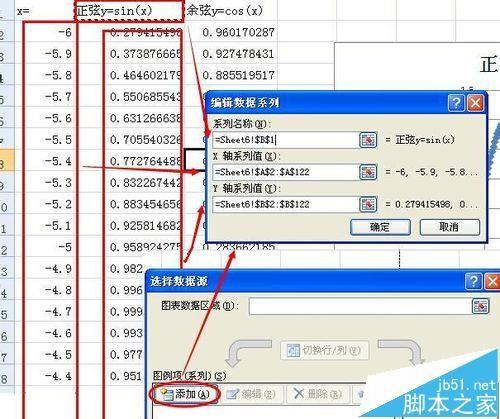5、添加了数据函数图像就出来了，如图，我们发现有好多地方不符合要求，需要修改的。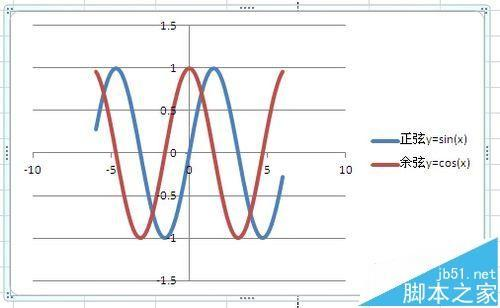6、首先图例和网格线，我们不需要，可以删除。选择图表中的图例，按Delete键删除，再选择网格线，按Delete键删除。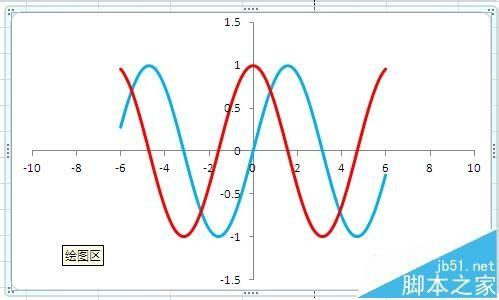7、其次两个坐标轴的刻度还不符合要求，为了更好的调整坐标轴，先要把图表区调成正方的，在“格式”选项卡“大小”组中，把高度和宽度调成一样的。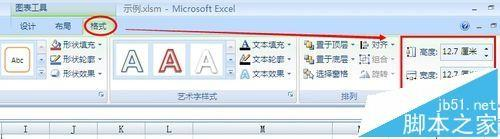8、鼠标在每一个坐标轴上右击，在弹出的快捷菜单中选择“设置坐标轴格式”。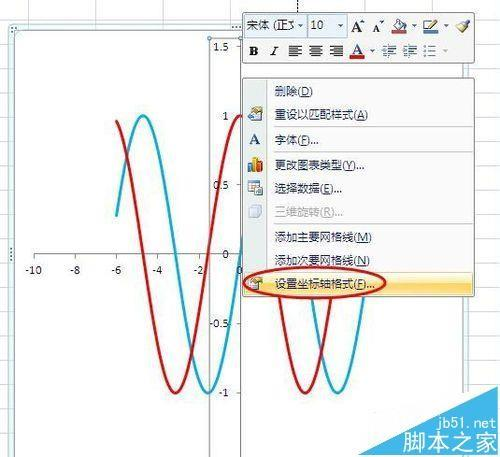9、在弹出的“设置坐标轴格式”对话框中的“坐标轴选项”栏目中，将“最小值”、“最大值”、“主要刻度单位”的值设置为“固定”，并在后面的文本框中分别输入“-6”、“6”、“1”，单击“关闭”按钮。两个轴进行同样的设置。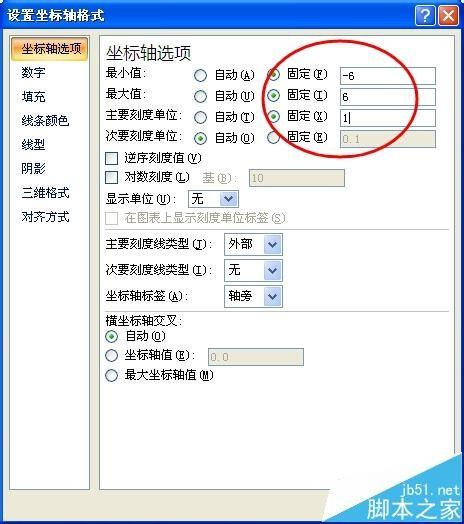10、然后，在设置一下函数图像的线条颜色和线型，鼠标在函数图像上右击，在弹出的快捷菜单中选择“设置数据系列格式”。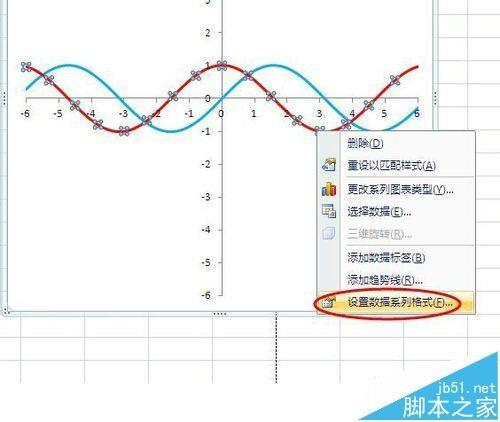11、在弹出的“设置数据系列格式”对话框中的“线条颜色”、“线型”栏目中，分别设置函数图像的线条颜色和线型。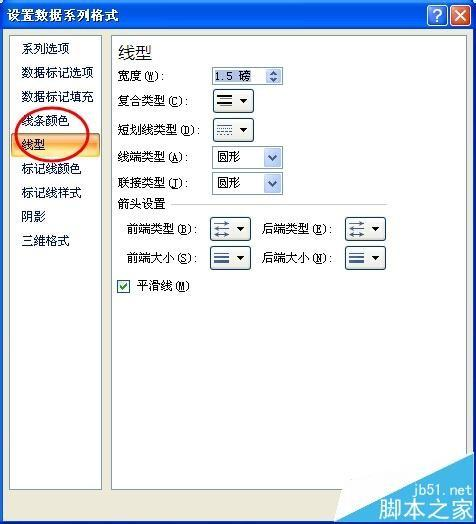12、最后给每个图像添加一个函数表达式，先单击选择一个函数图像，然后在一个数据点上单击选择一个数据点，再在数据点右击，在弹出的快捷菜单中选择“添加数据标签”。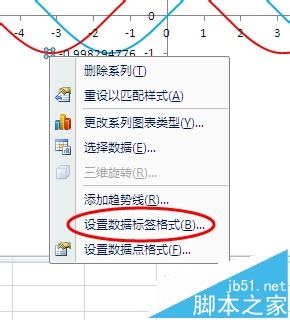13、然后再在数据点右击，在弹出的快捷菜单中选择“设置数据标签格式”。14、在弹出的“设置数据标签格式”对话框中，分别设置函数标签的内容、位置及边框颜色等。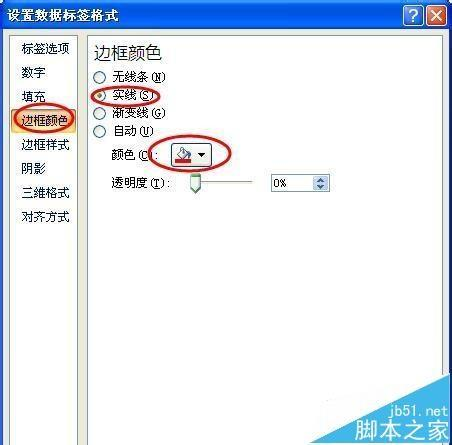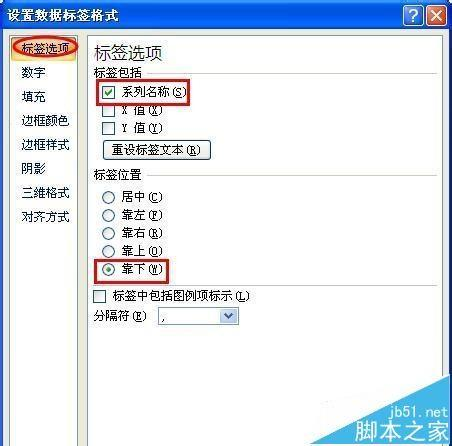15、到此既标准又漂亮的函数图像就设置完成了。下面是我作的抛物线函数图像。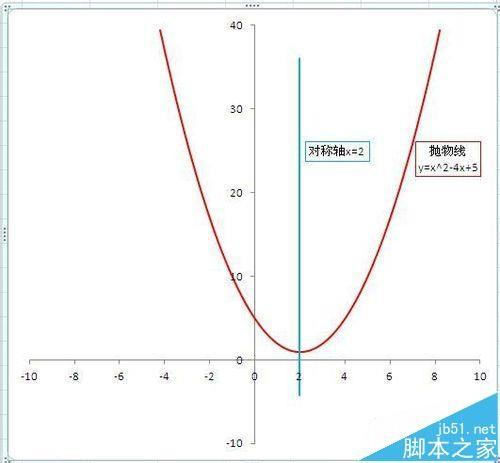展开全文• 语法：rand函数随机取100个从-1到1(原来写成了2)的数x1，x2，，x=rand(1,100)*2-1。扩展资料MATLAB和Mathematica、Maple并称三大数学软件。在数学类科技应用软件中在数值计算方面首屈一指。MATLAB可以进行矩阵...
• matlab画传递函数曲线

千次阅读 2021-05-07 07:26:34
如何matlab画开环传递函数的奈奎斯特图MATLAB...稳定性可根据奈奎斯特判据判定：开环系怎样MATLAB输入一个传递函数以G(s)=10/s(s+1)(s^2/4+1)例这种传递函数是零极点描述形式,因而要使用sys=zpk(z,p,k)的命...
• (2019年2月19日注：这篇文章原先发在自己github那边的博客，时间是2016年10月28日)最近应该是六叔的物化理论作业要交了吧，很多人问我六叔的作业里面有两道题要怎么进行图像函数的拟合。综合起来的问题主要有两个：1...
• 信息举报时间：2020-12-24 本页您甄选多篇描写函数图象关于y轴对称是什么函数,函数图象关于y轴对称是什么函数精选,函数图象关于y轴对称是什么函数大全，有议论，叙事 ，想象等形式。文章字数有400字、600字、800字...
• 如何在excel中绘制函数图像？图类型散点图。在任意数据点上，点右键。。 添加趋势线。。。。类型任选。。。在选项标签页里。。勾选下面显示公式。。。显示R值平方。。一定要是右键数据点，其它地方不会出来的。...
• 一个非常偶然的机会，我刚好出差中，手头电脑里没有安装Matlab，恰好此时又需要做一个曲线拟合的工作，于是我就找到了Plotly。先来看一眼Plotly能绘制怎样的专业图形：以上三幅图是plotly官方给出的示例，用户可以...
• 几何画板作为使用专业的几何绘图软件，自带动画演示功能，本节将介绍利用几何画板作正弦函数图象的方法。具体的操作步骤如下：步骤一 打开画板，建立直角坐标系(菜单栏里选择“绘图”——“定义坐标系”)，在空白处...java画函数图像
• TikZ可以画很多函数图像，例如一次函数，二次函数，三角函数，以及其他能够表达式表达的函数。使用的命令是：\draw[domain=参数：范围] plot(x,y)，其中domain指明了参数范围，冒号隔开。plot(f(x),f(y))指明...
• 函数图像

2021-06-28 13:04:47
具体而言，如果x实数，则函数图形在平面直角坐标系上呈现一条曲线。如果函数自变量x两个实数组成的有序对(x1, x2)，则图形就是所有三重序(x1, x2, f(x1, x2))组成的集合，呈现曲面(参见三维计算机图形)。...
• 函数曲线图是研究函数的重要工具。R 中 curve() 函数可以绘制函数图像，代码格式如下：curve(expr, from = NULL, to = NULL, n = 101, add = FALSE,type = "l", xname = "x", xlab = xname, ylab = NULL,log = ...
• 本文实例大家分享了Android九宫格图片展示的具体代码，供大家参考，具体内容如下题目：在屏幕上“*”显示0°~360°的余弦函数cos(x)曲线。#include #include int main(){int x;double y;int m;int i;for (y = 1;...
• fun 拟合曲线对应的函数，对应函数文件应保存在工作目录下、并针对所有数据点(因而输入数据在 fun 中定义)；x0 初始值(初始值一定要给fun中未知数x个数相等，即与返回拟合参数的个数相等，初始值可以任意给定，...
• 记住：原函数与反函数是关于y=x...对于正余弦函数图像可用五点作图法 以sinx例 五点：(0,0)(π/2,1)(π，0)(3π/2,-1)(2π，0) 以cosx例 五点：(0,1)(π/2,0)(π，-1)(3π/2,0)(2π，1) 而其.y=-2-cosx的定义域...
• 下面小编就教你怎么在WPS表格中绘制二次函数曲线图。WPS表格中绘制二次函数曲线图的方法：①启动WPS表格2013，先设定abc的值，也就是二次函数的三个系数。②我们知道，a是不能0的，所以，有必要设置数据有效性，设...
• 三次样条函数 去模拟汽车门的曲线，车门曲线的型值点数据如下0 1 2 3 4 5 6 7 8 9 102.51 3.30 4.04 4.70 5.22 5.54 5.78 ...
• np.cos(i*x)) plt.show() 使用Python画数学函数曲线 使用Python画数学函数曲线 import numpy as np import pandas as pd import matplotlib.pyplot as plt plt.figure(1) x = np.linspace(1, 200, 400) y=1000*(2.0-...
• 几何画板作为使用专业的几何绘图软件，自带动画演示功能，本节将介绍利用几何画板作正弦函数图象的方法。具体的操作步骤如下：步骤一 打开画板，建立直角坐标系(菜单栏里选择“绘图”——“定义坐标系”)，在空白处...
• 本文介绍一下使用R中curve函数绘制常见函数曲线的方法。1、curve函数简介curve函数语法格式如下：curve(expr, from = NULL, to = NULL, n = 101, add = FALSE,type = "l", xname = "x", xlab = xname, ylab = NULL,...
• 大的矩阵可以分行输入，回车键代替分号，这和我们平时使用的矩阵形式很相近。例如 a=[1 2 3 4 5 6] 大部分的试验数据使用上面的形式给出的，在处理试验数据中，可以简单的将数据前后加入左右括号，就可以得到矩阵...
• C语言sin()函数绘制正弦曲线代码（0-2π）

千次阅读 多人点赞 2020-12-27 12:32:38
本文实现y=sin(x)正弦曲线在0-2π范围内的曲线图形，如下图所示： 完整C语言代码： #include<stdio.h> #include<math.h> main() { double y; int x,m,i; printf("y=sin(x) [0<x<2*pi]\n...C语言 正弦曲线 sin 三角函数...